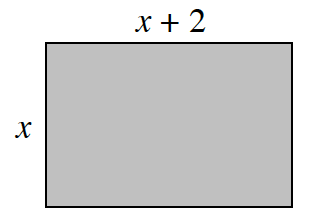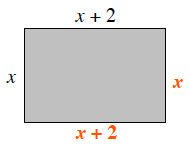Home > CC2 > Chapter 5 > Lesson 5.3.3 > Problem5-123

5-123.

If the total area of the rectangle below is 168 square units, how long is each side? To find out how long the x side must be, copy the diagram and table and answer the questions that follow. Homework Help ✎

Describe/Draw:Define Do Decide Side #1 Side #2 (Side one) · (Side two) $\text{Area}=168?$ Trial 1: $10$ $12$ Trial 2:

Declare:

1. Describe how the lengths of the two sides are related to each other.

Identify which side is longer and which is shorter. Label the value of the lengths of each side. Can you find a relationship between these values?2. Which side of the rectangle does Side #2 represent?

By looking at the side lengths in the chart, we see that Side #2 is greater than Side #1.

Side #2 represents the $x+2$ side of the tile shape.

3. Use the 5‑D Process to complete the table. Find the lengths of the two sides of the rectangle.

 Define Do Decide Side #1 Side #2 (Side one) · (Side two) $\text{Area}=168?$ Trial 1: $10$ $12$ $\mathbf{(10)(12)=120}$ Too Low Trial 2: $\mathbf{12}$ $\mathbf{14}$ $\mathbf{(12)(14)=168}$ Yes, this must be the correct answer.